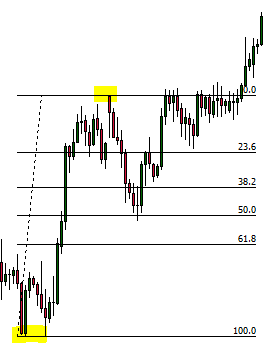top of page

# Forex 101 - Fibonacci Retracement

Leonardo Fibonacci was an Italian mathematician most known for the Fibonacci sequence. You really don't need to know the who, why, what and when of Leonardo Fibonacci to use Fibonacci to trade Forex. All you do need to know is that there is a very common trading tool/indicator that is named after him.

There are 2 main areas of trading with Fibonacci; Fibonacci retracement and Fibonacci extension. In this post, we will focus on Fibonacci retracement.

Fibonacci Retracement

Fibonacci retracement is a technical tool/indicator that is used to identify potential areas where a retracement or pull-back may end and where the next swing may begin. If you need a reminder about pushes and pull-backs (swings and retracements), please read from our Forex Price Action Basics - Trending Markets.

Below are 2 examples of the Fibonacci retracement tool. The first would be used for a bullish retracement, the second for a bearish retracement.The indicator marks 4 levels of potential reversal; 23.6%, 38.2%, 50.0% and 61.8%. The tool is drawn by using the high to low of the most recent swing low (if price is down-trending) or the low to high of the most recent swing high (if price is up-trending).

Below is an example of the Fibonacci retracement tool being drawn on a down-trend...Do you notice that the tool has been drawn from the top of the recent swing high to the tip of the recent swing low? Can you also see the Fib levels of 23.6, 38.2, 50.0 and 61.8 marked on the chart? These levels are potential areas where the retracement may reverse and price may attempt to swing lower - creating a new swing high. Let's have a look and see what happens...Price finds resistance and reverses bearish around the 61.8% level!

Below is an example of the Fibonacci retracement tool being draw on a up-trend. Can you see how the tool has been drawn using the high and low of the most recent swing high? Do you also see the Fib levels?This time price found support around the 50.0% level.

This is the basics of Fibonacci retracement. Just as with all technical indicators and drawings, Fibonacci retracement is not 100% reliable.

Fibonacci is Self-Fulfilling

The main reason why Fibonacci works when trading Forex is that it is self-fulfilling. Fibonacci is widely used amongst traders and investors. The Fib levels can be watched carefully and buy and sell orders can be placed around these levels - creating potential support and resistance.